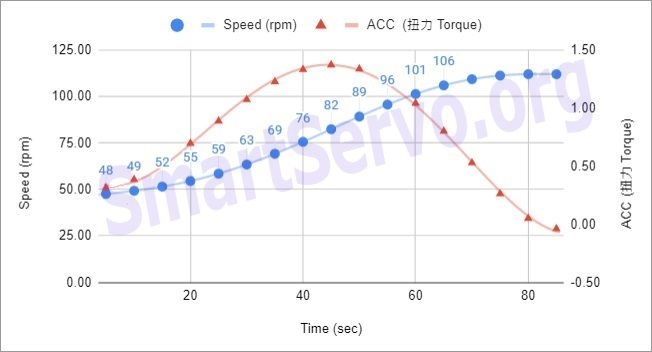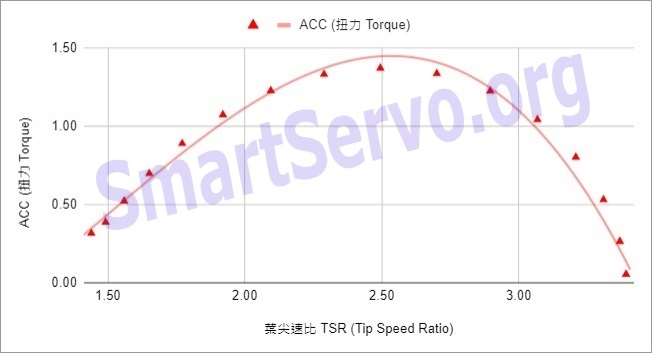# High-efficiency but difficult to self-start Darrieus wind turbine experiment – VAWT (2)

According to the literature, the Darrieus vertical axis wind turbine characterized by high efficiency but it is difficult to be self-starting. I couldn’t understand when I first came into contact with it. Since high efficiency means that it has torque, why can’t it start by itself? Later I realized that its efficiency must appear at a higher speed (similar to the high gear of a car), As for how high the speed should be? Usually 2~6 times faster than wind speed. This puzzles me even more, because the wind turbine is driven by the wind, why can it revolve faster than the wind speed? It seems that an experiment must be done, seeing is believing …

related video is as follows：

As you can see from the film, it is indeed difficult for the Darrieus wind turbine to be self-starting. Even if it gets a little initial velocity, the initial acceleration is very slow. Indicates that the torque at low speed is very small, which is very similar to the feeling of driving a car in fourth gear to start. As the speed increases, the acceleration becomes faster and faster. The maximum speed reached is 110 rpm, and the linear velocity of the blades calculated has reached 3.3 times the wind speed. It is indeed much faster than the wind ! (The wind speed was 1.8 m/sec and the radius of the wind turbine was 0.52 m)

Tip Speed Ratio (TSR) – Important parameter of wind turbine performance

Tip Speed Ratio (TSR) represents the ratio of blade linear speed to wind speed, the formula is as follows：

TSR$\lambda =\frac{V_{line}}{ V_\infty}=\frac{2\pi \times V_{rpm} \cdot R}{60\cdot V_\infty}$

where：$V_{line}$ ：blade linear speed(m/sec)$V_\infty$ ：wind speed(m/sec)$V_{rpm}$ ：revolution speed of wind turbine(revolutions per minute)$R$ ：Blade revolution radius(m)

Take the experimental data into the formula：

TSR$\lambda =\frac{2\pi \times 110 (rpm) \cdot 0.52}{60\cdot 1.8} =3.33$

Tip Speed Ratio (TSR) is an important parameter that affects wind turbine performance. The Darrieus wind turbine is a lift-type vertical axis wind turbine, and the angle of attack of the blades will change continuously when it revolves. Too small TSR will cause the angle of attack to change too much and cause the blade to stall. When the TSR is 3~6, the blade angle of attack is in a suitable range, and the blade lift is much greater than the drag, so high efficiency can be obtained. However, the TSR of the drag-type wind turbine cannot exceed 1, so the efficiency is low.

After the wind turbine in this experiment revolves, record its speed (rpm) and time (sec), and obtain the speed curve in Figure 1 through curve fitting. Then differentiate it to get the acceleration (ACC) curve, which is also plotted in Figure 1. The acceleration can also be regarded as the torque generated by the wind turbine.Figure 1: The speed and torque curves of the Darrieus wind turbine in this experiment

Then convert the wind turbine speed(rpm) in Figure 1 to tip speed ratio (TSR), and plot it with the corresponding torque as shown in Figure 2. It can be seen that the maximum torque of the wind turbine in this experiment appears when the tip speed ratio is Near 2.5.Figure 2: The torque v.s. TSR curve of the Darrieus wind turbine in this experiment

[註 1] The angle of attack is the angle between the blade chord line and the relative wind speed, which has a decisive influence on the performance of the lift blade. The angle of attack must be within a certain range (for example, +/- 10 degrees) to maintain a good lift-to-drag ratio. If the angle of attack is too large, the lift will disappear, which is called a stall.